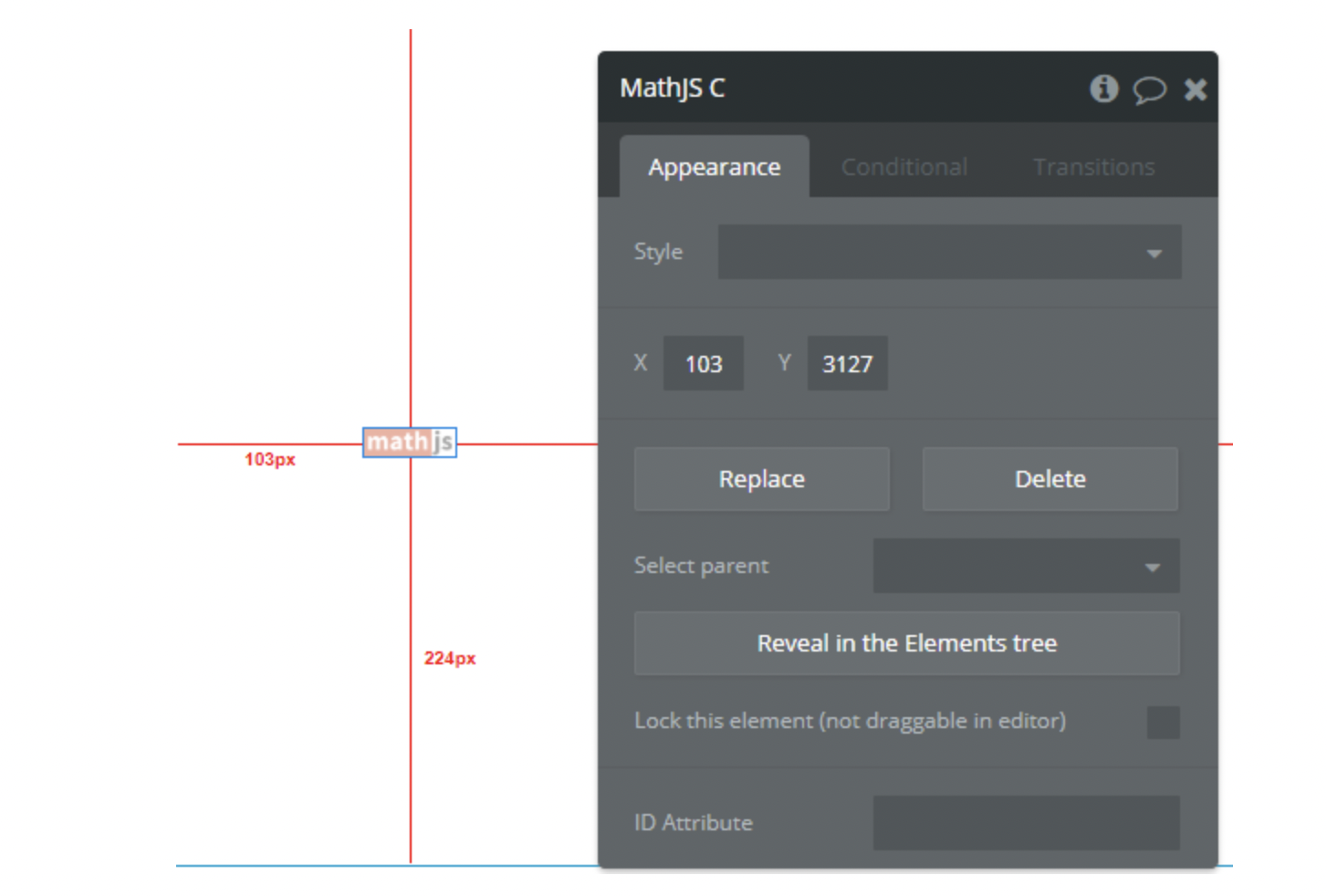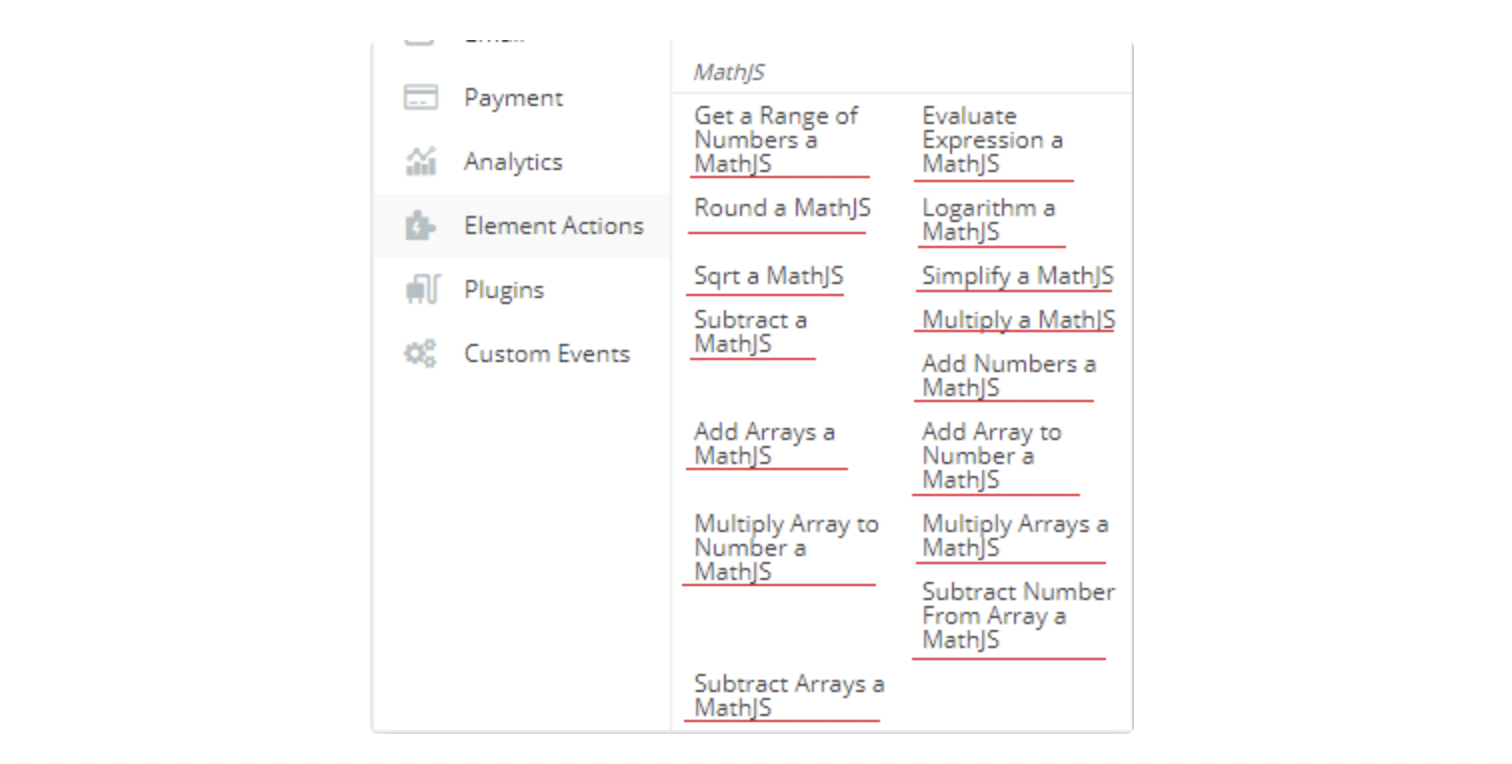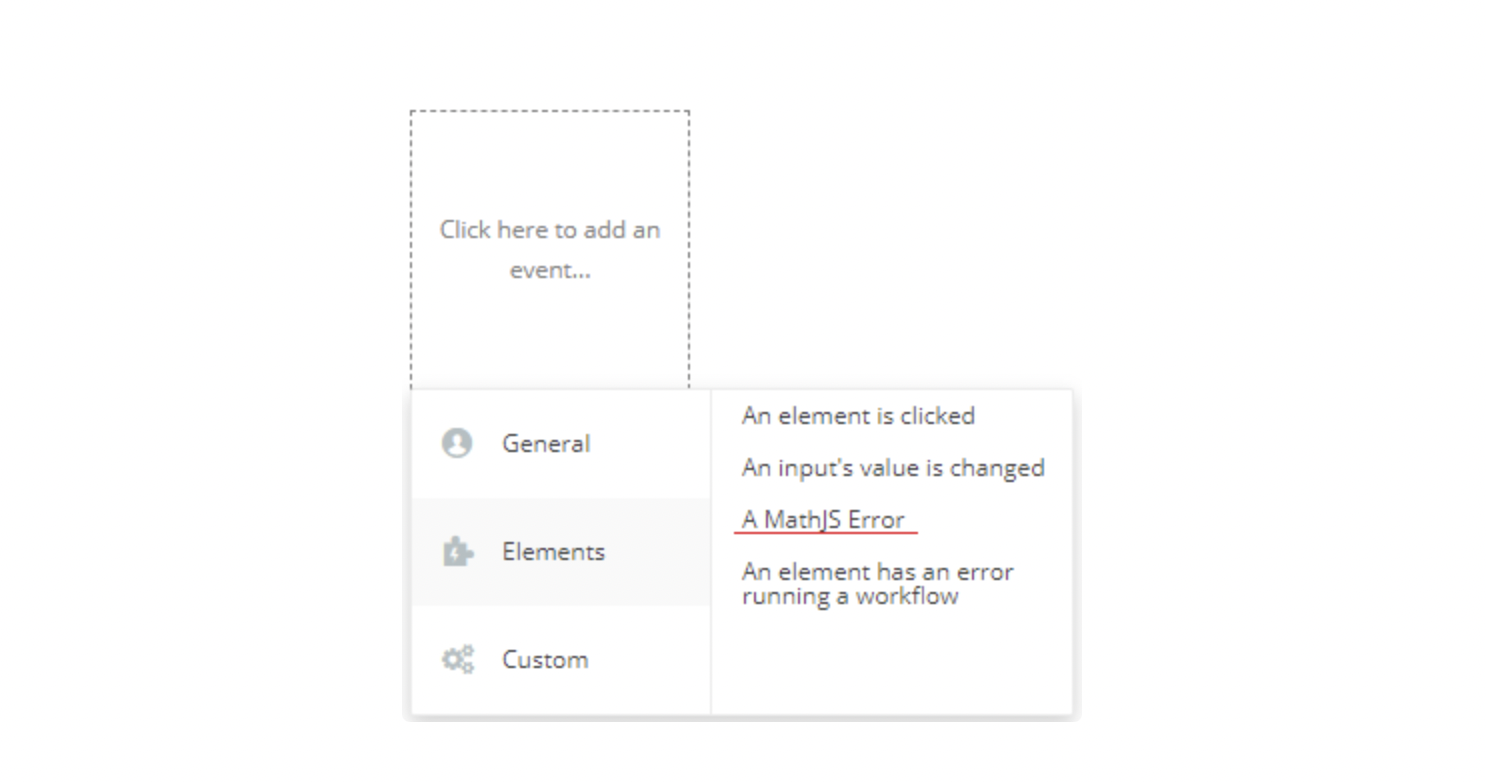12min

# OVERVIEW

Math.js is an extensive math library and features a flexible expression parser with support for symbolic computation, comes with a large set of built-in functions and constants, and offers an integrated solution to work with different data types like numbers, big numbers, complex numbers, fractions, units, strings, arrays and matrices. Powerful and easy to use.

PURPOSE

Setting up and using the mathematical powerful tool of math.js in inside your Bubble web application.

# Setting up

### Step 1. Install the math.js element on the Bubble page﻿

All the details on regarding the calculations, expressions can be found here: http://mathjs.org/

### Step 2. Choose the element actions to use plugin

In workflow now you can use Math.js’s actions to start using it in your application.﻿

Each action returns the result of calculations in form of its own state as described below:

• “Get a Range of Numbers” uses 2 input parameters, type number (bubble - number) and by making the operation, it returns the result in the “Range Result” state. The type of state is a matrice (bubble - list of numbers).

• “Evaluate Expression” uses as input parameter a mathematical expression (ex: “sin(45 deg) ^ 2”) and after making the calculations, it returns the result in “Evaluate Result” state. The type state is a number (bubble - number).

• “Round” uses 2 input parameters, type number (bubble - number), the first parameter value is a decimal and the second one is integer and by making the operation, it returns the result in the “Round Result” state. The type of the state is a number (bubble - number).

• “Logarithm” uses 2 input parameters, type number (bubble - number), the first parameter is the value for which we want to get the logarithm of and the second one is the base of the logarithm logbx and by making the operation, it returns the result in the “Logarithm Result” state. The type of the state is a number (bubble - number).

• “Sqrt” uses as input parameter, type number (bubble - number) and by making the operation, it returns the square root of the number (nth-root) in the “Sqrt Result” state. The type of the state is a string (bubble - text), the returned result is text due to user may choose to make square root from a negative number (ex. -4) with the result 2i.

• “Simplify” uses as input parameter a mathematical expression (ex: “x^2 + x + 3 + x^2”) and after making the calculations, it returns the result in the “Simplify Result” state. The type of the state is a string (bubble - text).

• “Subtract”, “Multiply”, “Add Numbers” use 2 input parameters, type number (bubble - number) and by making the operation, they return results in the respective state “Subtract Result”, “Multiply Result”, “Add Result”. The type of these states is a number (bubble - number).

• “Subtract Arrays”, “Add Arrays” use 2 input parameters, type 2 matrices with numbers (bubble - list of numbers) and by making the operation, they return results in the respective state “Subtract Arrays Result”, “Add Arrays Result”. The type of these state is a matrix (bubble - list of numbers).

• “Multiply Arrays” uses 2 input parameters, type 2 matrices with numbers (bubble - list of numbers) and by making the operation, it returns the result in the “Multiply Arrays Result” state. The type of the state is a number (bubble - number).

• “Add Array To Number”, “Multiply Array To Number”, “Subtract Number From Array” use 2 input parameters, one type matrix and second type number and after making the calculations, they return the results in “Add Array to Number Result”, “Multiply Array to Number Result”, “Subtract Number From Array Result”. These three states have the type a matrix (bubble - list of numbers).

Another thing besides these states, the plugin has an “error” state, which returns the result of errors if any appear for debugging, as well an “error” event which is triggered if the errors appear.

This event can be used as notification system for user in case if errors appear in calculations.# Demo to preview the settings

﻿

Updated 19 Apr 2022